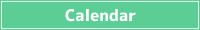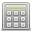<< < Dec. 2023 > >>
SunMonTueWedThuFriSat
26 27 28 29 30 1 2
3 4 5 6 7 8 9
10 11 12 13 14 15 16
17 18 19 20 21 22 23
24 25 26 27 28 29 30
31 1 2 3 4 5 6## Percentage Calculator

Calculations such as what percent of a certain number is, or X percent of a certain number are some.

What is the X percent of a number?

% of ?

What percent of the total is a certain number?

What % of is ?

When a number has X percent of the whole, what is the whole?

When % of the whole is , what is the total?

A ratio is a value that represents the amount of one source relative to another.

It expresses how much the amount compared to the standard occupies as a ratio.

So the ratio can be calculated by dividing the amount to be compared by the original amount to be compared.

Ratio = Amount compared / Original amount

Ratios are expressed as fractions, decimals, or percentages.

When expressed as a decimal or fraction, the ratio is less than 1 if the amount being compared is less than the underlying amount.

Conversely, if the quantity being compared is greater than the underlying quantity, the ratio will be greater than 1.

For example, if there are 20 boys in a class of 50, the ratio of boys to class is 20 / 50 = 0.4.

A percentage is a unit that expresses a percentage, and the whole is expressed as 100.

When expressing a ratio as a fraction or a decimal, the whole is expressed as 1, while the percentage is expressed as 100, so it can be calculated by multiplying by 100.

If there are 20 boys in the class of 50 people, the ratio of boys to the class is 20 / 50 × 100 = 40%.

It is based on 100, so if the compared quantity is less than the base quantity, it will be less than 100 percent, and if the compared quantity is greater than the base quantity, it will be greater than 100 percent.

## How to calculate percentage

### What is the X percent of a number?

If you want to calculate X percent of some number, you can do it with
Some Number × (Percent / 100)

Percentages represent the ratio when the whole is 100, so you can find the ratio when the whole is 1 by dividing the percentage by 100.

By multiplying a number by its percentage, you can calculate the X percent of a number.

### What percent of the total is a certain number?

If you want to calculate the percentage of a number, you can do it with
Some Number / Whole × 100

A ratio is a value that expresses the amount of something else in relation to a source amount, so you can find the ratio by dividing a number by the whole.

When expressed as a percentage, the whole is set to 100, so by multiplying the ratio by 100, you can calculate the percentage.

### Given a number with X percent, what is the total?

If you want to calculate some whole number when there is X percent, you can do it with
Some Number / Percent × 100

Since it is a number that has X percent of the whole, if you want to find the whole from a certain number, you can find it by dividing it by the ratio.

Since it's a percentage, you can calculate the whole by multiplying it by 100.

###Simple Calculator

Save results in a temporary